# Solving BPDN$_\infty$ for quantized Compressed sensing via the cvxopt package¶

$\newcommand{\eps}{\varepsilon}$ $\newcommand{\bR}{\mathbb{R}}$ $\newcommand{\bZ}{\mathbb{Z}}$ $\newcommand{\1}{{\rm 1}\kern-0.24em{\rm I}}$ $\newcommand{\inr}{\bigl< #1 \bigr>}$

This notebooks is a simulations support for Section~7 of paper

Sjoerd, Dirksen and Guillaume, Lecué and Holger, Rauhut, "ON THE GAP BETWEEN RIP-PROPERTIES AND SPARSE RECOVERY CONDITIONS"

We refer the reader to paper for more details

In :
from __future__ import division
import time
%pylab inline

Populating the interactive namespace from numpy and matplotlib


# Import cvxopt library for solving our optimization problem¶

In :
from cvxopt import solvers, matrix, spdiag, log, spmatrix, sparse # writting '%pylab inline' after those lines will cause trouble in the matrix method
solvers.options['show_progress'] = False


# Solving BPDN$_\infty$ via linear programming¶

Given a measurements matrix $A\in\bR^{m\times n}$ and $y=A\hat x + e$ where $||e||_\infty\leq \eps$, the Basis Pursuit Denoising program BPDN$_\infty$ is the procedure

$$min\big( ||t||_1: ||At-y||_\infty\leq \eps\big) \hspace{2cm} (P).$$

This procedure can be recast as a linear program by introducing slack variables $z^+,z^-\in\bR^n$: problem~(P) is equivalent to

$$\min_{z^+,z^-\in\bR^n} \sum_{j=1}^n z_i^+ + z_i^-$$

subject to $$-\eps\leq [A|-A]\left[\begin{array}{c} z^+\\ z^-\end{array}\right]-y\leq \eps$$

and $$\left[\begin{array}{c} z^+\\ z^-\end{array}\right]\geq 0$$

This is a linear program:

$$\min_{\left[\begin{array}{c} z^+\\ z^-\end{array}\right]\in\bR^{2n}} \inr{a,\left[\begin{array}{c} z^+\\ z^-\end{array}\right]}$$

subject to $$M \left[\begin{array}{c} z^+\\ z^-\end{array}\right] \leq b$$

where

$$a = \left[\begin{array}{c} \1 \\ \1 \end{array}\right],\hspace{1cm} M = \left[\begin{array}{c}[A|-A]\\ [-A|A]\\ [-I_n|0]\\ [0|-I_n] \end{array}\right],\hspace{1cm} b = \left[\begin{array}{c}y + \eps \1\\ -y+\eps\1 \\ 0 \\ 0 \end{array}\right]$$

Solution to (P) is recovered via $t= z^+-z^-$.

### Construction of cvxopt matrices $a,M,b$¶

Given the measurement matrix $A\in\bR^{m\times n}$, the vector of measures $y\in\bR^m$ and a $\ell_\infty$ bound $\eps$ on the noise, we construct cvxopt matrices $a,M,b$ as in the Linear Programming formulation of BPDN$_\infty$.

In :
def cvx_mat(A, y, eps):
'''A, y: numpy array or cvx matrices
eps : positive real number'''
A = matrix(A)
y = matrix(y)
m, n = matrix(A).size
# matrix a
a = matrix(ones(2*n))
# matrix M
I_n = spdiag(*n)
z_n = spdiag(*n)
M = matrix([[A,-A, -I_n, z_n],[-A, A, z_n, -I_n]])
# matrix b
un_m = matrix(ones(m))
zero_n = matrix(zeros(n))
b = matrix([y + eps*un_m, -y + eps*un_m, zero_n, zero_n])
return a, M, b


### Construction of sparse signals in $\bR^n$ and noisy measurements in $\bR^m$¶

We construct signals with sparsity given by sparsity, with a randomly chosen support in $\{1,\ldots,n\}$ and with none zero coefficients equal to $1$.

We construct two types of measures:

1. measures are obtained by $y_i= \inr{A_{i,\cdot},signal} + e$ where $e$ is a variable chosen randomly in $[-\eps,\eps]$
2. measures are obtained by quantization; that is $y_i$ is equal to the closest point in $\eps\bZ$ of $\inr{A_{i,\cdot},signal}$
In :
def signal(n, sparsity):
sel = random.permutation(n)
sel = sel[0:sparsity]   # indices of the nonzero elements of xsharp
xsharp = zeros(n)
xsharp[sel] = 1
return xsharp

def measures(A, signal, eps):
m, n = A.shape
e = random.uniform(-eps, eps, (m,1))
return [sum(a) for a in zip(dot(A, signal), e)]

def measures_quantized(A, signal, eps):
y = dot(A, signal)
if eps>0:
y_q = [int(ele/eps)*eps for ele in y]
else:
y_q = y
return y_q


### cvxopt linear solver cvx lp ¶

#### First try exact reconstruction by setting $\eps=0$¶

In :
m , n, sparsity, eps = 15, 50, 3, 0
A, x_hat = randn(m, n), signal(n, sparsity)
y = measures(A, x_hat, eps)

In :
a, M, b = cvx_mat(A, y, eps)
#print(a, M, b)

In :
sol = solvers.lp(a, M, b)
sol = sol['x']
x_recover = sol[0:n] - sol[n:2*n]

In :
norm(matrix(x_hat) - x_recover,2)

Out:
4.0640307445255899e-08

#### Then try reconstruction from noisy measurements (i.e. noise level $\eps>0$)¶

In :
m , n, sparsity, eps = 10, 40, 3, 0.1
A, x_hat = randn(m, n), signal(n, sparsity)


random noise

In :
y = measures(A, x_hat, eps)
a, M, b = cvx_mat(A, y, eps)

In :
sol = solvers.lp(a, M, b)
sol = sol['x']
x_recover = sol[0:n] - sol[n:2*n]
norm(matrix(x_hat) - x_recover,2)

Out:
1.1840669138367335

quantized measurements

In :
y_quant = measures_quantized(A, x_hat, eps)
a, M, b = cvx_mat(A, y_quant, eps)

In :
sol = solvers.lp(a, M, b)
sol = sol['x']
x_recover = sol[0:n] - sol[n:2*n]
norm(matrix(x_hat) - x_recover,2)

Out:
1.2310718900162763

# Phase transition diagram for BPDN$_\infty$¶

We construct two types of phase diagram:

1. One where the number of success is counted. We say that the reconstruction is a success when $||x_{hat}-x_{recover}||_2\leq 20\eps + 0.001$ (where signal = $x_{hat}$)
2. One where the errors $||x_{hat}-x_{recover}||_2$ are added for every pixel of the phase transition matrix (we don't have to decide wheither it is a succes or a failure)

#### We start with a phase transition diagram where successes are counted; and for random uniform noise¶

In :
def dist(x_hat, sol):
n = len(x_hat)
x_recover = sol[0:n] - sol[n:2*n]
return norm(matrix(x_hat) - x_recover,2)

In :
def phase_transition_mat(n, eps, nbtest):
"""return a n.n/2 matrix with the number of reconstruction success for every  1\leq m \leq n measurements
and sparsity 1\leq sparsity \leq n/2
n : ambiant dimension of the signals
eps : infinite norm of the additive noise (= twice the size of cells in CS quantization)
nbtest : number of tests for each pixel"""
PTM = zeros((n,int(n/2)))
for m in range(1,n+1):#construct one line of the Phase transition matrix for a given number of measurements m
if (m % 20) == 0:
print("line number {} done".format(m))
A = randn(m,n) / sqrt(m)
for sparsity in range(1,min(m+1, int(n/2))+1):
nb_success = 0
for i in range(nbtest):
x_hat = signal(n, sparsity)
y = measures(A, x_hat, eps)
a, M, b = cvx_mat(A, y, eps)
sol = solvers.lp(a, M, b)
sol = sol['x']
if dist(x_hat, sol) <= 20*eps+0.001:
nb_success = nb_success + 1
PTM[m-1, sparsity-1] = nb_success
return PTM

In :
def frontier(mat, nbtest):
"""construction of the phase transition frontier, i.e. first time the number of success goes below nbtest/2"""
L = []
n = len(mat)
for s in range(int(n/2)):
m = 0
while mat[m,s]<nbtest/2 and m<n-1:
m = m + 1
L.append(m)
return L

In :
n, eps, nbtest = 60, 0, 10
#solvers.options['show_progress'] = False # No logs printed
mat = phase_transition_mat(n, eps, nbtest)# construction of the matrix with the number of success among nbtest

line number 20 done
line number 40 done
line number 60 done

In :
def mat_plot(mat, n, nbtest, titre):
P_min, P_max, S_min, S_max = 0, n, 0, int(n/2)-1
fig = plt.imshow(mat[P_min:P_max, S_min:S_max], interpolation="gaussian",
aspect='auto', origin = 'lower', extent=[S_min, S_max, P_min, P_max])
plt.title(titre)
plt.xlabel('sparsity')
plt.ylabel('number of measurements')

#empirical phase transition
X = range(int(n/2))
L = frontier(mat, nbtest)
plot(X,L, linewidth=4, color = 'black', label='phase transition')
plt.legend(loc=4)

In :
titre = "Gaussian measurements"
mat_plot(mat, n, nbtest, titre)
#filename = 'noisy_gaussian_{}_eps_{}.png'.format(n, eps)
#plt.savefig(filename,bbox_inches='tight')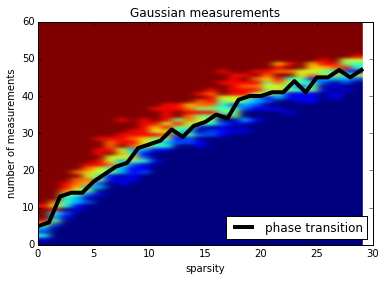#### Now, we plot the phase transition where the reconstruction errors are added.¶

We explore both random noise and quantized measurements

In :
def phase_transition_mat_sum_rand(n, eps, nbtest):
"""return a n.n/2 matrix with the sum of reconstruction errors at every pixel for every  1\leq m \leq n measurements
and sparsity 1\leq sparsity \leq n/2
n : ambiant dimension of the signals
eps : infinite norm of the additive noise (= twice the size of cells in CS quantization)
nbtest : number of tests for each pixel"""
PTM = zeros((n,int(n/2)))
for m in range(1,n+1):#construct one line of the Phase transition matrix for a given number of measurements m
if (m % 20) == 0:
print("line number {} done".format(m))
A = randn(m,n) / sqrt(m)
ind_failure = 0
for sparsity in range(1, int(n/2)+1):
sum_errors = 0
for i in range(nbtest):
x_hat = signal(n, sparsity)
y = measures(A, x_hat, eps)
a, M, b = cvx_mat(A, y, eps)
sol = solvers.lp(a, M, b)
sol = sol['x']
sum_errors = sum_errors + dist(x_hat, sol)
PTM[m-1, sparsity-1] = sum_errors
return PTM

In :
def frontier_sum(mat, eps, nbtest):
"""construction of the phase transition frontier, i.e. first time the number of success goes below nbtest/2"""
L = []
n = len(mat)
for s in range(int(n/2)):
m = 0
while mat[m,s]> nbtest*(20*eps+1) and m<n-1:
m = m + 1
L.append(m)
return L

In :
def mat_plot_sum(mat, n, titre):
P_min, P_max, S_min, S_max = 0, n, 0, int(n/2)-1
fig = plt.imshow(mat[P_min:P_max, S_min:S_max], interpolation="gaussian",
aspect='auto', origin = 'lower', extent=[S_min, S_max+1, P_min, P_max])
plt.title(titre)
plt.xlabel('sparsity')
plt.ylabel('number of measurements')

#empirical phase transition
X = range(int(n/2))
L = frontier_sum(mat, eps, nbtest)
plot(X,L, linewidth=4, color = 'black', label='phase transition')
plt.legend(loc=4)

In :
n, eps, nbtest = 80, 0.1, 10
start = time.time()
mat = phase_transition_mat_sum_rand(n, eps, nbtest)
print('{} seconds'.format(time.time() - start))

line number 20 done
line number 40 done
line number 60 done
line number 80 done
284.347899199 seconds

In :
titre = "Gaussian measurements -- random uniform noise (level = {})".format(eps)
mat_plot_sum(mat, n, titre)
#filename = 'Gaussian_random_noise_n_{}_eps_{}.png'.format(n, eps)
#plt.savefig(filename,bbox_inches='tight')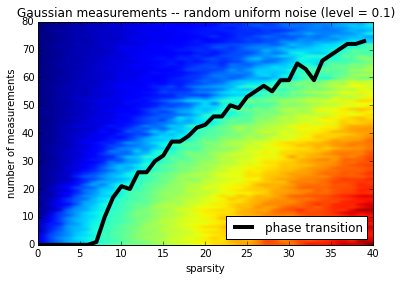#### now for quantized measurements¶

In :
def phase_transition_mat_sum_quant(n, eps, nbtest):
"""return a n.n/2 matrix with the sum of reconstruction errors at every pixel for every  1\leq m \leq n measurements
and sparsity 1\leq sparsity \leq n/2
n : ambiant dimension of the signals
eps : size of bins in CS quantization
nbtest : number of tests for each pixel"""
PTM = zeros((n,int(n/2)))
for m in range(1,n+1):#construct one line of the Phase transition matrix for a given number of measurements m
if (m % 20) == 0:
print("line number {} done".format(m))
A = randn(m,n) / sqrt(m)
for sparsity in range(1, int(n/2)+1):
sum_errors = 0
for i in range(nbtest):
x_hat = signal(n, sparsity)
y = measures_quantized(A, x_hat, eps)
a, M, b = cvx_mat(A, y, eps)
sol = solvers.lp(a, M, b)
sol = sol['x']
sum_errors = sum_errors + dist(x_hat, sol)
PTM[m-1, sparsity-1] = sum_errors
return PTM

In :
n, eps, nbtest = 60, 0.1, 10
start = time.time()
mat = phase_transition_mat_sum_quant(n, eps, nbtest)
print('{} seconds'.format(time.time()-start))

line number 20 done
line number 40 done
line number 60 done
101.018029213 seconds

In :
titre = "Gaussian measurements"
mat_plot_sum(mat, n, titre)
#filename = 'quantized_cs_gaussian_measures_n_{}_eps_{}.png'.format(n, eps)
#plt.savefig(filename,bbox_inches='tight')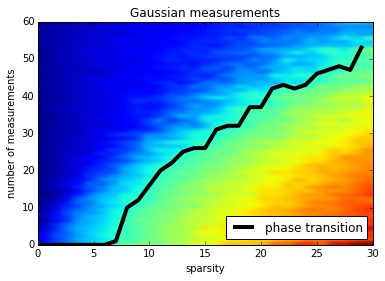### Repeat the construction of the frontier nb_curves times to "smooth it"¶

nb_curves: number of phase transition curves constructed. Those curves are then averaged to "smooth" the effect of randomness in phase transition and get a "stable" phase transition

In :
n, eps, nbtest, nb_curves = 40, 0.1, 10, 5
L = zeros(int(n/2))
start = time.time()
for i in range(nb_curves):
if (i % 10) == 0:
print('step {} done'.format(i))
mat = phase_transition_mat_sum_quant(n, eps, nbtest)
F = frontier_sum(mat, eps, nbtest)
L = [sum(a) for a in zip(L,F)]
L_gauss = [i/nb_curves for i in L]
print('{} seconds'.format(time.time()-start))

step 0 done
line number 20 done
line number 40 done
line number 20 done
line number 40 done
line number 20 done
line number 40 done
line number 20 done
line number 40 done
line number 20 done
line number 40 done
155.107507944 seconds

In :
#For next use, save the Gaussian phase transition frontier with pickle
#import pickle
#filename = 'L_gauss_n_{}_eps_{}.p'.format(n, eps)

#Save L_gauss
#with open(filename, 'wb') as fp:
#    pickle.dump(L_gauss, fp)

#filename = 'L_gauss_100_eps_0.1.p'
#with open(filename, 'rb') as fp:


### Draw the phase transition frontier for quantized CS for Gaussian measurements¶

(it took 38664.542408 seconds to get it for n, eps, nbtest, nb_curves = 100, 0.1, 15, 40)

In :
X = range(len(L_gauss))
plot(X,L_gauss, linewidth=4, color = 'blue', label="Gaussian phase transition")
titre = "Quantized CS - Gaussian measurements"
plt.title(titre)
plt.xlabel('sparsity')
plt.ylabel('number of measurements')
plt.legend(loc=4)
#filename = 'gaussian_phase_transition_quantized_cs_{}_eps_{}.png'.format(n, eps)
#plt.savefig(filename,bbox_inches='tight')

Out:
<matplotlib.legend.Legend at 0x108b5c1d0>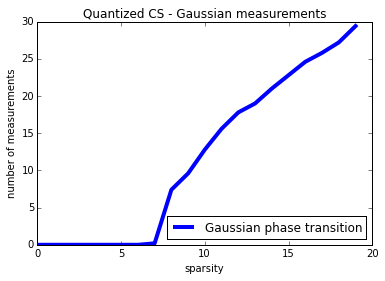### Phase transition curves for $\Psi_\alpha$ variables¶

In :
def mat_exp_power(m, n, alpha):
A = randn(m, n)/ sqrt(m)
return np.multiply(np.power(np.absolute(A),int(2/alpha)), sign(A))

In :
def phase_transition_mat_sum_quant_exp_power(n, eps, nbtest, alpha):
PTM = zeros((n,int(n/2)))
for m in range(1,n+1):#construct one line of the Phase transition matrix for a given number of measurements m
if (m % 20) == 0:
print("line number {} done".format(m))
A =  mat_exp_power(m, n, alpha)
for sparsity in range(1, int(n/2)+1):
sum_errors = 0
for i in range(nbtest):
x_hat = signal(n, sparsity)
y = measures_quantized(A, x_hat, eps)
a, M, b = cvx_mat(A, y, eps)
sol = solvers.lp(a, M, b)
sol = sol['x']
sum_errors = sum_errors + dist(x_hat, sol)
PTM[m-1, sparsity-1] = sum_errors
return PTM

In :
n, eps, nbtest, nb_curves = 50, 0.1, 10, 5
L = zeros(int(n/2))
dict_power_quant_cs = {2: L, 1.5: L, 1: L, 0.5: L}# note that for alpha<0.5 cvxopt solver fails
#dict_power_quant_cs = {2: L, 1.8: L, 1.5: L, 1.3: L, 1: L, 0.8: L, 0.5: L}
for alpha in dict_power_quant_cs.keys():
print('exp power {} running'.format(alpha))
for i in range(nb_curves):
mat = phase_transition_mat_sum_quant_exp_power(n, eps, nbtest, alpha)
F = frontier_sum(mat, eps, nbtest)
dict_power_quant_cs[alpha] = [sum(a) for a in zip(dict_power_quant_cs[alpha], F)]
dict_power_quant_cs[alpha] = [ele/nb_curves for ele in dict_power_quant_cs[alpha]]

exp power 1.5 running
line number 20 done
line number 40 done
line number 20 done
line number 40 done
line number 20 done
line number 40 done
line number 20 done
line number 40 done
line number 20 done
line number 40 done
exp power 1 running
line number 20 done
line number 40 done
line number 20 done
line number 40 done
line number 20 done
line number 40 done
line number 20 done
line number 40 done
line number 20 done
line number 40 done
exp power 2 running
line number 20 done
line number 40 done
line number 20 done
line number 40 done
line number 20 done
line number 40 done
line number 20 done
line number 40 done
line number 20 done
line number 40 done
exp power 0.5 running
line number 20 done
line number 40 done
line number 20 done
line number 40 done
line number 20 done
line number 40 done
line number 20 done
line number 40 done
line number 20 done
line number 40 done

In :
#save the dictionary of phase transitions curves to speed up later investigation
#import pickle
#filename = 'dictionnaries_quantized_cs_power_n_{}_eps_{}.p'.format(n, eps)
#with open(filename, 'wb') as fp:
#    pickle.dump(dict_power_quant_cs, fp)

#with open(filename, 'rb') as fp:

In :
X = range(int(n/2))
plt.figure(figsize=(8,6))
for key in dict_power_quant_cs.keys():
if key !=2:
L = dict_power_quant_cs[key]
text = 'exp power {}'.format(key)
plot(X, L, label = text)
plt.xlabel('sparsity')
plt.ylabel('number of measurements')
plt.title('phase transition curves - quantized CS - exponential moments')
#Gaussian phase transition
#n_gauss = len(L_gauss)
#X_gauss = range(n_gauss)
#plot(X_gauss, L_gauss, 'r--', linewidth=3, label="Gaussian phase transition")
#plot(X, L_gauss[0:int(n/2)], 'r--', linewidth=3, label="Gaussian phase transition")
#plt.legend(loc=4)
#filename = "phase_transition_curves_quant_cs_n_{}_eps_{}.png".format(n, eps)
#plt.savefig(filename, bbox_inches='tight')

Out:
<matplotlib.text.Text at 0x10d3caa50>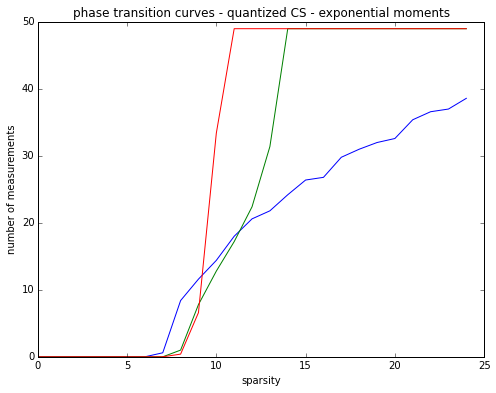### Phase transition curves for Student variables¶

In :
def mat_power(m, n, p):
return random.standard_t(p, size=(m, n))

In :
def phase_transition_mat_sum_quant_student(n, eps, nbtest, p):
PTM = zeros((n,int(n/2)))
for m in range(1,n+1):#construct one line of the Phase transition matrix for a given number of measurements m
if (m % 20) == 0:
print("line number {} done".format(m))
A =  mat_power(m, n, p)
for sparsity in range(1, int(n/2)+1):
sum_errors = 0
for i in range(nbtest):
x_hat = signal(n, sparsity)
y = measures_quantized(A, x_hat, eps)
a, M, b = cvx_mat(A, y, eps)
sol = solvers.lp(a, M, b)
sol = sol['x']
sum_errors = sum_errors + dist(x_hat, sol)
PTM[m-1, sparsity-1] = sum_errors
return PTM

In :
n, eps, nbtest, nb_curves = 40, 0.1, 10, 5
L = zeros(int(n/2))
#dict_quant_cs_student = {30: L, 20: L, 15: L, 10: L, 5: L, 4: L, 3: L, 2: L}
dict_quant_cs_student = {30: L, 20: L}
for p in dict_quant_cs_student.keys():
print('power {} running'.format(p))
for i in range(nb_curves):
mat = phase_transition_mat_sum_quant_student(n, eps, nbtest, p)
F = frontier_sum(mat, eps, nbtest)
dict_quant_cs_student[p] = [sum(a) for a in zip(dict_quant_cs_student[p], F)]
dict_quant_cs_student[p] = [ele/nb_curves for ele in dict_quant_cs_student[p]]

power 20 running
line number 20 done
line number 40 done
line number 20 done
line number 40 done
line number 20 done
line number 40 done
line number 20 done
line number 40 done
line number 20 done
line number 40 done
power 30 running
line number 20 done
line number 40 done
line number 20 done
line number 40 done
line number 20 done
line number 40 done
line number 20 done
line number 40 done
line number 20 done
line number 40 done

In :
#save the dictionary of phase transitions curves to speed up later investigation
#import pickle
#filename = 'dictionnaries_quantized_cs_student_n_{}_eps_{}.p'.format(n, eps)
#with open(filename, 'wb') as fp:
#    pickle.dump(dict_quant_cs_student, fp)

#with open(filename, 'rb') as fp:

In :
X = range(int(n/2))
plt.figure(figsize=(8,6))
for key in dict_quant_cs_student.keys():
if key != 15:
L = dict_quant_cs_student[key]
text = 'degree {}'.format(key)
plot(X, L, label = text)
plt.xlabel('sparsity')
plt.ylabel('number of measurements')
plt.title('phase transition curves - quantized CS - Student variables')
#Gaussian phase transition
#n_gauss = len(L_gauss)
#X_gauss = range(n_gauss)
plot(X, L_gauss[0:int(n/2)], 'r--', linewidth=3, label="Gaussian phase transition")
plt.legend(loc=4)
#filename = "phase_transition_curves_quant_cs_student_n_{}_eps_{}.png".format(n, eps)
#plt.savefig(filename, bbox_inches='tight')

Out:
<matplotlib.legend.Legend at 0x11310a1d0>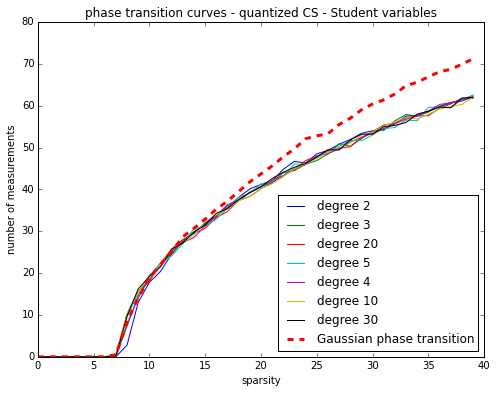# Reconstruction quality of BPDN$_\infty$ for large $n=1024$¶

Construction of phase transition diagrams may take a very long time in high dimensions (large values of $n$). That is the reason why we did not explore values of $n$ larger than $80$ (which is not high-dimensional).

In this last section, we explore a larger dimension $n=1024$. We consider the same simulation setup as the one in paper Dequantizing Compressed sensing from L. Jacques, D.K. Hammond and J.M. Fadili:

1. $$SNR(signal, signal_{reconstruct}) = 20*\log_{10}\Big(\frac{||signal||_2}{||signal-signal_{reconstruct}||}_2\Big)$$
2. eps : size of bins in CS quantization equal to ||A signal||_\infty/40
3. $n=1024$, sparsity = 16, nbtest = 500
4. A coefficient is satisfying QC when $$|(A signal_{reconstruct})_i-y_i|\leq eps/2$$
In :
def signal_gauss(n, sparsity):
sel = random.permutation(n)
sel = sel[0:sparsity]
x = zeros(n)
x[sel] = randn(sparsity)
return x

def SNR(x_hat, sol):
n = len(x_hat)
x_recover = sol[0:n] - sol[n:2*n]
return 20*log10(norm(x_hat,2)/norm(matrix(x_hat) - x_recover,2))

def QC(A, y, sol, eps):
minus_x_recover = sol[n:2*n] - sol[0:n]
pred = dot(A, minus_x_recover)
list_QC = [abs(sum(ele)) <= eps/2 for ele in zip(pred, y)]
return sum(list_QC)/len(y)

In :
def SNR_gauss_quant_cs(n, sparsity, nbtest, list_ratio):
"""Return a list of the average SNR(x_hat, sol) for different ratio m/sparsity
n : ambiant dimension of the signals
sparsity : sparsity of signal
nbtest : number of tests for point"""
list_SNR = []
list_SNR_std = []
list_QC = []
list_measures = [ele*sparsity for ele in list_ratio]
for m in list_measures:
print("measurement {} running".format(m))
A = randn(m,n)
sum_snr = 0
sum_snr_square = 0
sum_QC = 0
for i in range(nbtest):
x_hat = signal_gauss(n, sparsity)
eps = float(max(abs(dot(A,x_hat)))/40)
y = measures_quantized(A, x_hat, eps)
a, M, b = cvx_mat(A, y, eps)
sol = solvers.lp(a, M, b)
sol = sol['x']
sum_snr = sum_snr + SNR(x_hat, sol)
sum_snr_square = sum_snr_square + SNR(x_hat, sol)**2
sum_QC = sum_QC + QC(A, y, sol, eps)
list_SNR.append(sum_snr/nbtest)
list_SNR_std.append(sqrt(sum_snr_square/nbtest-(sum_snr/nbtest)**2))
list_QC.append(sum_QC/nbtest)
return list_SNR, list_SNR_std, list_QC

In :
n, sparsity, nbtest, list_ratio = 1024, 16, 100, [10, 15 , 20, 25, 30, 35 , 40]
start = time.time()
list_SNR_gauss, list_SNR_std_gauss, list_QC_gauss = SNR_gauss_quant_cs(n, sparsity, nbtest, list_ratio)
print('{} seconds'.format(time.time()-start))

measurement 160 running
measurement 240 running
measurement 320 running
measurement 400 running
measurement 480 running
measurement 560 running
measurement 640 running
3509.82822204 seconds

In :
import pickle
#list_SNR_gauss_quant_cs = [list_SNR_gauss, list_SNR_std_gauss, list_QC_gauss]
#filename = 'snr_quant_gauss_n_{}_sparsity_{}_nbtest_{}.p'.format(n, sparsity, nbtest)
#with open(filename, 'wb') as fp:
#    pickle.dump(list_SNR_gauss_quant_cs, fp)

#filename = 'snr_quant_gauss_n_1024_sparsity_16_nbtest.p'
with open(filename, 'rb') as fp:
list_SNR_gauss = list_SNR_gauss_quant_cs
list_SNR_std_gauss = list_SNR_gauss_quant_cs
list_QC_gauss = list_SNR_gauss_quant_cs

In :
#plot(list_ratio, list_SNR_gauss, color='blue', marker='*', markersize=15, label='Gaussian measurements')#marker='^', 'o', '8', 'H', '+', 'x'
#plt.legend(loc = 4)
#plt.xlabel('m/s')
#plt.ylabel('SNR (dB)')
#plt.title('Quality of BPDN$\infty$ - quantized CS - Gaussian measurements')
#filename = 'snr_quant_gauss_n_{}_sparsity_{}.png'.format(n, sparsity)
#plt.savefig(filename, bbox_inches='tight')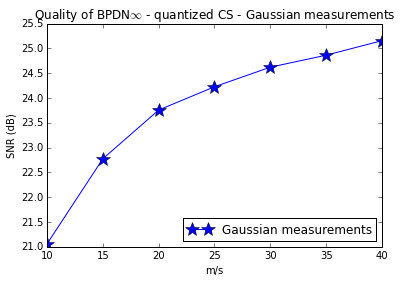In :
#plot(list_ratio, list_SNR_std_gauss, color='blue', marker='*', markersize=15, label='Gaussian measurements')
#plt.legend(loc = 4)
#plt.xlabel('m/s')
#plt.ylabel('SNR (dB)')
#plt.title('Standard deviation SNR of BPDN$\infty$')
#plt.legend(loc = 1)
#filename = 'snr_std_quant_gauss_n_{}_sparsity_{}.png'.format(n, sparsity)
#plt.savefig(filename, bbox_inches='tight')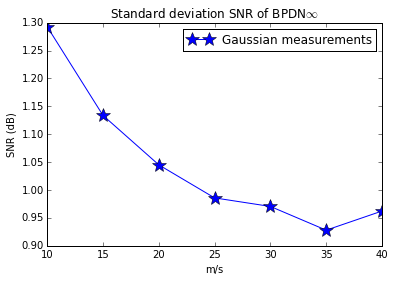In :
#plot(list_ratio, list_QC_gauss, color='blue', marker='*', markersize=15, label='Gaussian measurements')
#plt.xlabel('m/s')
#plt.ylabel('Average QC fraction')
#plt.title('QC fraction of BPDN$\infty$ -- Gaussian measurements')
#plt.legend(loc = 4)
#filename = 'snr_QC_quant_gauss_n_{}_sparsity_{}.png'.format(n, sparsity)
#plt.savefig(filename, bbox_inches='tight')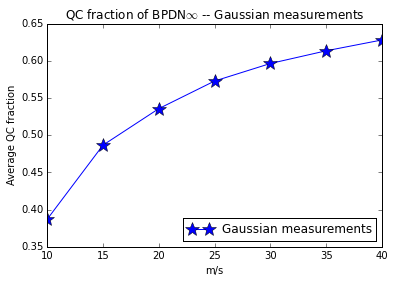In :
plt.figure(figsize=(15, 5))
subplot(131)
plot(list_ratio, list_SNR_gauss, color='blue', marker='*', markersize=15, label='Gaussian measurements')#marker='^', 'o', '8', 'H', '+', 'x'
plt.xlabel('m/s')
plt.ylabel('SNR (dB)')
plt.title('mean SNR')
plt.legend(loc = 4)
subplot(132)
plot(list_ratio, list_SNR_std_gauss, color='blue', marker='*', markersize=15, label='Gaussian measurements')#marker='^', 'o', '8', 'H', '+', 'x'
plt.xlabel('m/s')
plt.ylabel('SNR (dB)')
plt.title('standard deviation SNR')
subplot(133)
plot(list_ratio, list_QC_gauss, color='blue', marker='*', markersize=15, label='Gaussian measurements')
plt.xlabel('m/s')
plt.ylabel('Average QC fraction')
plt.title('Fraction of coefficient satisfying QC')
#filename = 'snr_subplots_quant_gauss_n_{}_sparsity_{}.png'.format(n, sparsity)
#plt.savefig(filename, bbox_inches='tight')

Out:
<matplotlib.text.Text at 0x10d57eb90>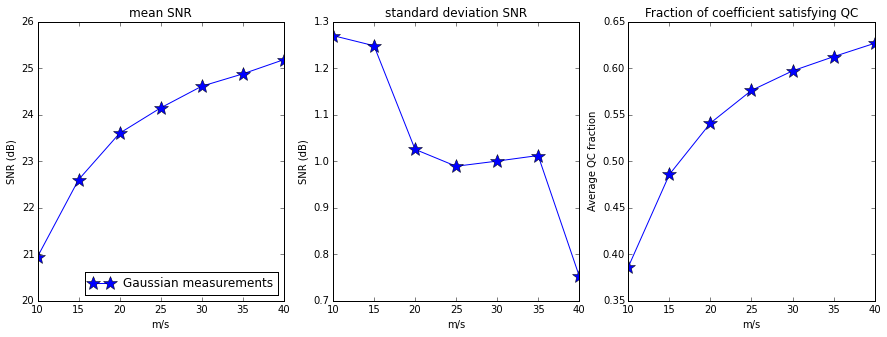#### Histogram of residuals for Gaussian measurements ($m=40*sparsity$)¶

In :
def SNR_gauss_quant_cs_hist(n, sparsity, nbtest):
"""Return a list of the (normalized) prediction error (residuals) (A signal_reconstruct-y)_i
n : ambiant dimension of the signals
sparsity : sparsity of signal
nbtest : number of tests for point"""
m = 40*sparsity
list_residue_avg = zeros(m)
A = randn(m,n)/sqrt(m)
for i in range(nbtest):
x_hat = signal_gauss(n, sparsity)
eps = float(max(abs(dot(A,x_hat)))/40)
y = measures_quantized(A, x_hat, eps)
a, M, b = cvx_mat(A, y, eps)
sol = solvers.lp(a, M, b)
sol = sol['x']
minus_x_recover = sol[n:2*n] - sol[0:n]
pred = dot(A, minus_x_recover)
list_residue = [sum(ele)/eps for ele in zip(pred, y)]
list_residue_avg = list_residue_avg +  list_residue
return list_residue_avg

In :
n, sparsity, nbtest = 100, 4, 10#1024, 16, 100
start = time.time()
list_hist = SNR_gauss_quant_cs_hist(n, sparsity, nbtest)
print('{} seconds'.format(time.time()-start))

0.229198932648 seconds

In :
#filename = "list_hist_gauss_n_{}_sparsity_{}_nbtest_{}.p".format(n, sparsity, nbtest)
#with open(filename, 'wb') as fp:
#    pickle.dump(list_hist, fp)

#with open(filename, 'rb') as fp:

In :
plt.hist(list_hist)#, bins=100, normed=True)
plt.axvline(x = 1/2)#, 'r--')#, linewidth=4)
plt.axvline(x = -1/2)#, 'r--')#, linewidth=4)
#filename = "list_hist_gauss_n_{}_sparsity_{}_nbtest_{}.png".format(n, sparsity, nbtest)
#plt.savefig(filename, bbox_inches='tight')

Out:
<matplotlib.lines.Line2D at 0x1998e22d0>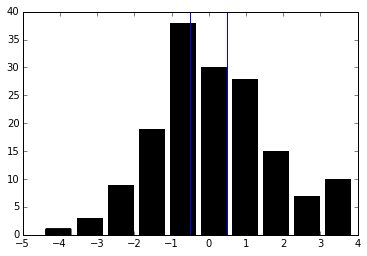### SNR for exponential power measurements matrices¶

In :
def mat_exp_power(m, n, alpha):
A = randn(m, n)/ sqrt(m)
return np.multiply(np.power(np.absolute(A),int(2/alpha)), sign(A))

In :
def SNR_exp_power_quant_cs(n, sparsity, nbtest, list_ratio, list_exp_power):
"""Return a list of the average SNR(x_hat, sol) for different ratio m/sparsity
n : ambiant dimension of the signals
sparsity : sparsity of signal
nbtest : number of tests for point"""
dict_SNR_exp_power = {}
list_measures = [ele*sparsity for ele in list_ratio]
for alpha in list_exp_power:
print("----- power {} running".format(alpha))
list_SNR = []
list_SNR_std = []
list_QC = []
for m in list_measures:
print("measurement {} running".format(m))
A = mat_exp_power(m, n, alpha)
sum_snr = 0
sum_snr_square = 0
sum_QC = 0
for i in range(nbtest):
x_hat = signal_gauss(n, sparsity)
eps = float(max(abs(dot(A,x_hat)))/40)
y = measures_quantized(A, x_hat, eps)
a, M, b = cvx_mat(A, y, eps)
sol = solvers.lp(a, M, b)
sol = sol['x']
sum_snr = sum_snr + SNR(x_hat, sol)
sum_snr_square = sum_snr_square + SNR(x_hat, sol)**2
sum_QC = sum_QC + QC(A, y, sol, eps)
list_SNR.append(sum_snr/nbtest)
list_SNR_std.append(sqrt(sum_snr_square/nbtest-(sum_snr/nbtest)**2))
list_QC.append(sum_QC/nbtest)
dict_SNR_exp_power[alpha] = [list_SNR, list_SNR_std, list_QC]
return dict_SNR_exp_power

In :
n, sparsity, nbtest, list_ratio, list_exp_power = 1024, 16, 100, [10, 15 , 20, 25, 30, 35 , 40], [2, 1.5, 1, 0.5]
dict_SNR_exp_power = SNR_exp_power_quant_cs(n, sparsity, nbtest, list_ratio, list_exp_power)

----- power 2 running
measurement 160 running
measurement 240 running
measurement 320 running
measurement 400 running
measurement 480 running
measurement 560 running
measurement 640 running
----- power 1.5 running
measurement 160 running
measurement 240 running
measurement 320 running
measurement 400 running
measurement 480 running
measurement 560 running
measurement 640 running
----- power 1 running
measurement 160 running
measurement 240 running
measurement 320 running
measurement 400 running
measurement 480 running
measurement 560 running
measurement 640 running
----- power 0.5 running
measurement 160 running
measurement 240 running
measurement 320 running
measurement 400 running
measurement 480 running
measurement 560 running
measurement 640 running

In :
#import pickle
#filename = "dict_SNR_exp_power_n_{}_sparsity_{}_nbtest_{}.p".format(n, sparsity, nbtest)
#with open(filename, 'wb') as fp:
#    pickle.dump(dict_SNR_exp_power, fp)

#with open(filename, 'rb') as fp:

In :
marker = ['v', '+', '.', 'o', '*']
plt.figure(figsize=(15, 5))
subplot(131)
ind = 0
for key in dict_SNR_exp_power.keys():
L = dict_SNR_exp_power[key]
text = 'power {}'.format(key)
plot(list_ratio, L, marker = marker[ind], markersize=5, label=text)
ind = ind + 1
plt.xlabel('m/s')
plt.ylabel('SNR (dB)')
plt.title('mean SNR')
plt.legend(loc = 4)
subplot(132)
ind = 0
for key in dict_SNR_exp_power.keys():
L = dict_SNR_exp_power[key]
text = 'power {}'.format(key)
plot(list_ratio, L, marker = marker[ind], markersize=5, label=text)
ind+=1
plt.xlabel('m/s')
plt.ylabel('SNR (dB)')
plt.title('standard deviation SNR')
subplot(133)
ind = 0
for key in dict_SNR_exp_power.keys():
L = dict_SNR_exp_power[key]
text = 'power {}'.format(key)
plot(list_ratio, L,  marker = marker[ind], markersize=5, label=text)
ind+=1
plt.xlabel('m/s')
plt.ylabel('Average QC fraction')
plt.title('Fraction of coefficient satisfying QC')
#filename = 'snr_subplots_quant_exp_power_n_{}_sparsity_{}_nbtest_{}.png'.format(n, sparsity, nbtest)
#plt.savefig(filename, bbox_inches='tight')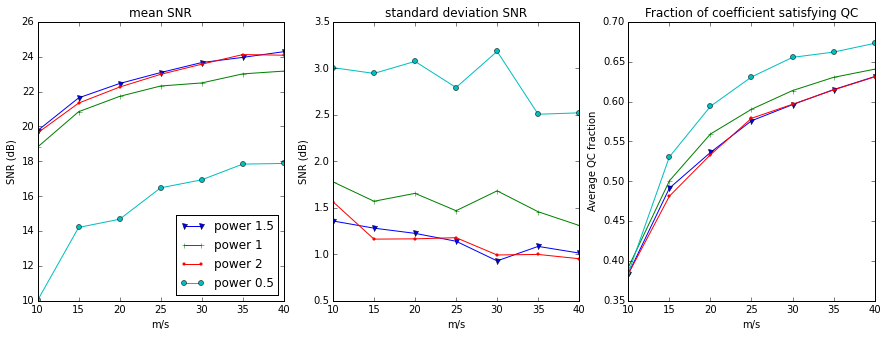### SNR for Student measurements matrices¶

In :
def mat_power(m, n, p):
return random.standard_t(p, size=(m, n))

In :
def SNR_student_quant_cs(n, sparsity, nbtest, list_ratio, list_degrees):
"""Return a list of the average SNR(x_hat, sol) for different ratio m/sparsity
n : ambiant dimension of the signals
sparsity : sparsity of signal
nbtest : number of tests for each point"""
dict_SNR_student = {}
list_measures = [ele*sparsity for ele in list_ratio]
start = time.time()
for p in list_degrees:
print("---- degree {} running -- {} seconds".format(p, time.time()-start))
list_SNR = []
list_SNR_std = []
list_QC = []
for m in list_measures:
print("measurement {} running".format(m))
A = mat_power(m, n, p)
sum_snr = 0
sum_snr_square = 0
sum_QC = 0
for i in range(nbtest):
x_hat = signal_gauss(n, sparsity)
eps = float(max(abs(dot(A,x_hat)))/40)
y = measures_quantized(A, x_hat, eps)
a, M, b = cvx_mat(A, y, eps)
sol = solvers.lp(a, M, b)
sol = sol['x']
sum_snr = sum_snr + SNR(x_hat, sol)
sum_snr_square = sum_snr_square + SNR(x_hat, sol)**2
sum_QC = sum_QC + QC(A, y, sol, eps)
list_SNR.append(sum_snr/nbtest)
list_SNR_std.append(sqrt(sum_snr_square/nbtest-(sum_snr/nbtest)**2))
list_QC.append(sum_QC/nbtest)
dict_SNR_student[p] = [list_SNR, list_SNR_std, list_QC]
return dict_SNR_student

In :
n, sparsity, nbtest, list_ratio, list_degrees = 1024, 16, 200, [10, 15 , 20, 25, 30, 35 , 40], [2, 3, 5, 10, 20]
dict_SNR_student = SNR_student_quant_cs(n, sparsity, nbtest, list_ratio, list_degrees)

---- degree 2 running -- 9.53674316406e-07 seconds
measurement 160 running
measurement 240 running
measurement 320 running
measurement 400 running
measurement 480 running
measurement 560 running
measurement 640 running
---- degree 3 running -- 6223.41036606 seconds
measurement 160 running
measurement 240 running
measurement 320 running
measurement 400 running
measurement 480 running
measurement 560 running
measurement 640 running
---- degree 5 running -- 12929.707541 seconds
measurement 160 running
measurement 240 running
measurement 320 running
measurement 400 running
measurement 480 running
measurement 560 running
measurement 640 running
---- degree 10 running -- 19866.689492 seconds
measurement 160 running
measurement 240 running
measurement 320 running
measurement 400 running
measurement 480 running
measurement 560 running
measurement 640 running
---- degree 20 running -- 26878.2539561 seconds
measurement 160 running
measurement 240 running
measurement 320 running
measurement 400 running
measurement 480 running
measurement 560 running
measurement 640 running

In :
#import pickle
#filename = "dict_SNR_student_n_{}_sparsity_{}_nbtest_{}.p".format(n, sparsity, nbtest)
#with open(filename, 'wb') as fp:
#    pickle.dump(dict_SNR_student, fp)

#with open(filename, 'rb') as fp:

In :
plt.figure(figsize=(15, 5))
marker = ['v', '+', '.', 'o', '*']
subplot(131)
ind = 0
for key in dict_SNR_student.keys():
L = dict_SNR_student[key]
text = 'degree {}'.format(key)
plot(list_ratio, L, marker = marker[ind], label=text)
ind+=1
plot(list_ratio, list_SNR_gauss , label='Gaussian measures')
plt.xlabel('m/s')
plt.ylabel('SNR (dB)')
plt.title('mean SNR')
subplot(132)
ind = 0
for key in dict_SNR_student.keys():
L = dict_SNR_student[key]
text = 'degree {}'.format(key)
plot(list_ratio, L, marker = marker[ind], label=text)
ind+=1
plot(list_ratio, list_SNR_std_gauss , label='Gaussian measures')
plt.xlabel('m/s')
plt.ylabel('SNR (dB)')
plt.title('standard deviation SNR')
subplot(133)
ind = 0
for key in dict_SNR_student.keys():
L = dict_SNR_student[key]
text = 'degree {}'.format(key)
plot(list_ratio, L, marker = marker[ind], label=text)
ind+=1
plot(list_ratio, list_QC_gauss , label='Gaussian measures')
plt.xlabel('m/s')
plt.ylabel('Average QC fraction')
plt.title('Fraction of coefficient satisfying QC')
plt.legend(loc = 4)
filename = 'snr_subplots_quant_student__n_{}_sparsity_{}.png'.format(n, sparsity)
plt.savefig(filename, bbox_inches='tight')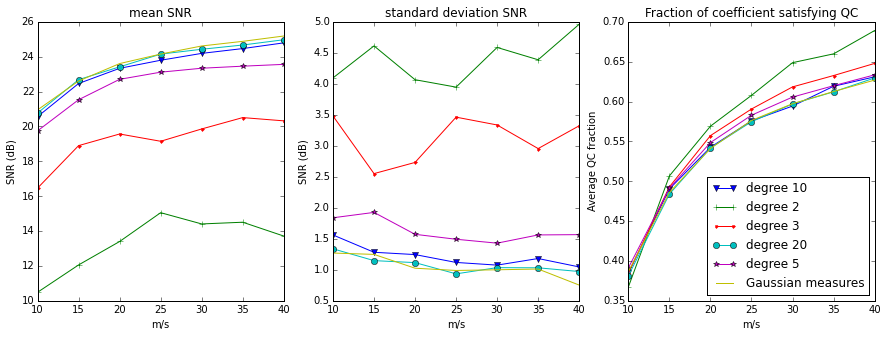### histogram of residuals for Student variables¶

In [ ]:
def mat_power(m, n, p):
return random.standard_t(p, size=(m, n))

In [ ]:
def SNR_student_quant_cs_hist(n, sparsity, nbtest, student_degree):
"""Return a list of the (normalized) prediction error (residuals) (A signal_reconstruct-y)_i
n : ambiant dimension of the signals
sparsity : sparsity of signal
nbtest : number of tests for point"""
m = 40*sparsity
list_residue_avg = zeros(m)
A = mat_power(m, n, student_degree)
for i in range(nbtest):
x_hat = signal_gauss(n, sparsity)
eps = float(max(abs(dot(A,x_hat)))/40)
y = measures_quantized(A, x_hat, eps)
a, M, b = cvx_mat(A, y, eps)
sol = solvers.lp(a, M, b)
sol = sol['x']
minus_x_recover = sol[n:2*n] - sol[0:n]
pred = dot(A, minus_x_recover)
list_residue = [sum(ele)/eps for ele in zip(pred, y)]
list_residue_avg = [sum(ele) for ele in zip(list_residue_avg, list_residue)]
return [ele/nbtest for ele in list_residue_avg]

In [ ]:
n, sparsity, nbtest, student_degree = 100, 5, 10, 4
start = time.time()
list_student_hist = SNR_student_quant_cs_hist(n, sparsity, nbtest, student_degree)
print('{} seconds'.format(time.time()-start))

In [ ]:
#filename = "list_hist_student_n_{}_sparsity_{}_nbtest_{}_degree_{}.p".format(n, sparsity, nbtest, student_degree)
#with open(filename, 'wb') as fp:
#    pickle.dump(list_student_hist, fp)

#with open(filename, 'rb') as fp:

In [ ]:
plt.hist(list_student_hist, bins=40, normed=True)
axvline(x = 1/2, linewidth=4, 'r--')
axvline(x = -1/2, linewidth=4,'r--')

#filename = "list_hist_student_n_{}_sparsity_{}_nbtest_{}_degree_{}.png".format(n, sparsity, nbtest, student_degree)
#plt.savefig(filename, bbox_inches='tight')


# Phase transition diagrams for correlated measurements¶

In this last section, we consider gaussian vectors with correlated entries as measurements vectors.

In :
def covariance_mat(n, p):
"""covariance matrix with off-diagonal coefficients equal p and diagonal coefficients equal 0"""
return (1-p)*identity(n) + p*ones((n,n))

def mat_gauss_corr(n, m, p):
mean = zeros(n)
cov = covariance_mat(n,p)
return random.multivariate_normal(mean, cov, m)

In :
def phase_transition_mat_sum_quant_gauss_corr(n, eps, nbtest, p):
PTM = zeros((n,int(n/2)))
for m in range(1,n+1):#construct one line of the Phase transition matrix for a given number of measurements m
if (m % 20) == 0:
print("line number {} done".format(m))
A =  mat_gauss_corr(n, m, p)
for sparsity in range(1, int(n/2)+1):
sum_errors = 0
for i in range(nbtest):
x_hat = signal(n, sparsity)
y = measures_quantized(A, x_hat, eps)
a, M, b = cvx_mat(A, y, eps)
sol = solvers.lp(a, M, b)
sol = sol['x']
sum_errors = sum_errors + dist(x_hat, sol)
PTM[m-1, sparsity-1] = sum_errors
return PTM

In :
n, eps, nbtest, nb_curves = 100, 0.1, 10, 10
L = zeros(int(n/2))
dict_gauss_corr_quant_cs = {0: L, 0.05: L, 0.1: L, 0.2: L}# note that for alpha<0.5 cvxopt solver fails
#dict_power_quant_cs = {2: L, 1.8: L, 1.5: L, 1.3: L, 1: L, 0.8: L, 0.5: L}
for p in dict_gauss_corr_quant_cs.keys():
print('correlation parameter {} running'.format(p))
for i in range(nb_curves):
mat = phase_transition_mat_sum_quant_gauss_corr(n, eps, nbtest, p)
F = frontier_sum(mat, eps, nbtest)
dict_gauss_corr_quant_cs[p] = [sum(a) for a in zip(dict_gauss_corr_quant_cs[p], F)]
dict_gauss_corr_quant_cs[p] = [ele/nb_curves for ele in dict_gauss_corr_quant_cs[p]]

correlation parameter 0 running
line number 20 done
line number 40 done
line number 60 done
line number 80 done
line number 100 done
line number 20 done
line number 40 done
line number 60 done
line number 80 done
line number 100 done
line number 20 done
line number 40 done
line number 60 done
line number 80 done
line number 100 done
line number 20 done
line number 40 done
line number 60 done
line number 80 done
line number 100 done
line number 20 done
line number 40 done
line number 60 done
line number 80 done
line number 100 done
line number 20 done
line number 40 done
line number 60 done
line number 80 done
line number 100 done
line number 20 done
line number 40 done
line number 60 done
line number 80 done
line number 100 done
line number 20 done
line number 40 done
line number 60 done
line number 80 done
line number 100 done
line number 20 done
line number 40 done
line number 60 done
line number 80 done
line number 100 done
line number 20 done
line number 40 done
line number 60 done
line number 80 done
line number 100 done
correlation parameter 0.05 running
line number 20 done
line number 40 done
line number 60 done
line number 80 done
line number 100 done
line number 20 done
line number 40 done
line number 60 done
line number 80 done
line number 100 done
line number 20 done
line number 40 done
line number 60 done
line number 80 done
line number 100 done
line number 20 done
line number 40 done
line number 60 done
line number 80 done
line number 100 done
line number 20 done
line number 40 done
line number 60 done
line number 80 done
line number 100 done
line number 20 done
line number 40 done
line number 60 done
line number 80 done
line number 100 done
line number 20 done
line number 40 done
line number 60 done
line number 80 done
line number 100 done
line number 20 done
line number 40 done
line number 60 done
line number 80 done
line number 100 done
line number 20 done
line number 40 done
line number 60 done
line number 80 done
line number 100 done
line number 20 done
line number 40 done
line number 60 done
line number 80 done
line number 100 done
correlation parameter 0.2 running
line number 20 done
line number 40 done
line number 60 done
line number 80 done
line number 100 done
line number 20 done
line number 40 done
line number 60 done
line number 80 done
line number 100 done
line number 20 done
line number 40 done
line number 60 done
line number 80 done
line number 100 done
line number 20 done
line number 40 done
line number 60 done
line number 80 done
line number 100 done
line number 20 done
line number 40 done
line number 60 done
line number 80 done
line number 100 done
line number 20 done
line number 40 done
line number 60 done
line number 80 done
line number 100 done
line number 20 done
line number 40 done
line number 60 done
line number 80 done
line number 100 done
line number 20 done
line number 40 done
line number 60 done
line number 80 done
line number 100 done
line number 20 done
line number 40 done
line number 60 done
line number 80 done
line number 100 done
line number 20 done
line number 40 done
line number 60 done
line number 80 done
line number 100 done
correlation parameter 0.1 running
line number 20 done
line number 40 done
line number 60 done
line number 80 done
line number 100 done
line number 20 done
line number 40 done
line number 60 done
line number 80 done
line number 100 done
line number 20 done
line number 40 done
line number 60 done
line number 80 done
line number 100 done
line number 20 done
line number 40 done
line number 60 done
line number 80 done
line number 100 done
line number 20 done
line number 40 done
line number 60 done
line number 80 done
line number 100 done
line number 20 done
line number 40 done
line number 60 done
line number 80 done
line number 100 done
line number 20 done
line number 40 done
line number 60 done
line number 80 done
line number 100 done
line number 20 done
line number 40 done
line number 60 done
line number 80 done
line number 100 done
line number 20 done
line number 40 done
line number 60 done
line number 80 done
line number 100 done
line number 20 done
line number 40 done
line number 60 done
line number 80 done
line number 100 done

In :
#save the dictionary of phase transitions curves to speed up later investigation
#import pickle
#filename = 'dictionnaries_quantized_cs_gauss_corr_n_{}_eps_{}.p'.format(n, eps)
#with open(filename, 'wb') as fp:
#    pickle.dump(dict_gauss_corr_quant_cs, fp)

#with open(filename, 'rb') as fp:

In :
X = range(int(n/2))
plt.figure(figsize=(8,6))
for key in sort(list(dict_gauss_corr_quant_cs.keys())):
L = dict_gauss_corr_quant_cs[key]
text = 'p = {}'.format(key)
plot(X, L, label = text)
plt.xlabel('sparsity')
plt.ylabel('number of measurements')
plt.title('phase transition curves - quantized CS - correlated measurements')
#Gaussian phase transition
#n_gauss = len(L_gauss)
#X_gauss = range(n_gauss)
#plot(X_gauss, L_gauss, 'r--', linewidth=3, label="Gaussian phase transition")
#plot(X, L_gauss[0:int(n/2)], 'r--', linewidth=3, label="Gaussian phase transition")
plt.legend(loc=4)
filename = "phase_transition_curves_quant_cs_gauss_corr_n_{}_eps_{}.png".format(n, eps)
plt.savefig(filename, bbox_inches='tight')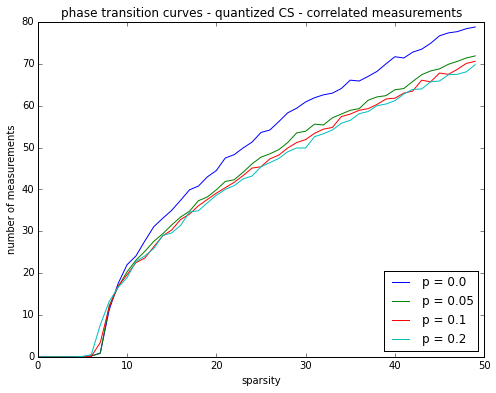In [ ]: